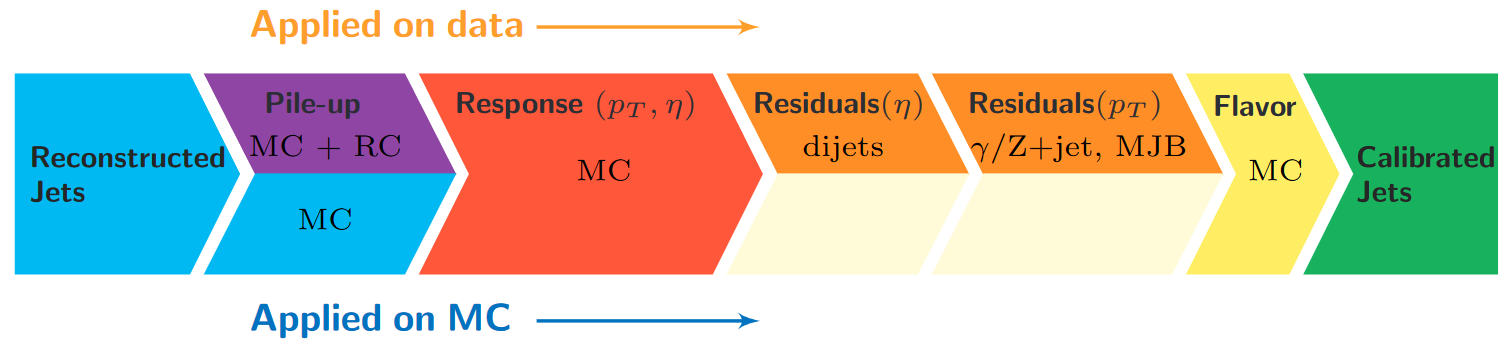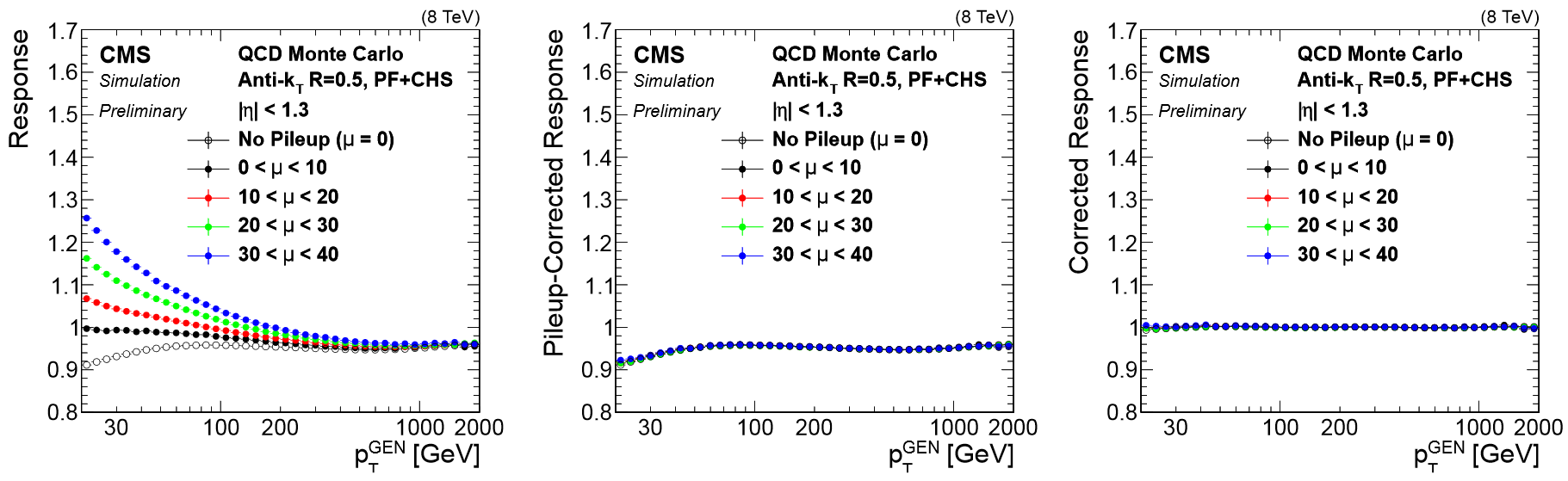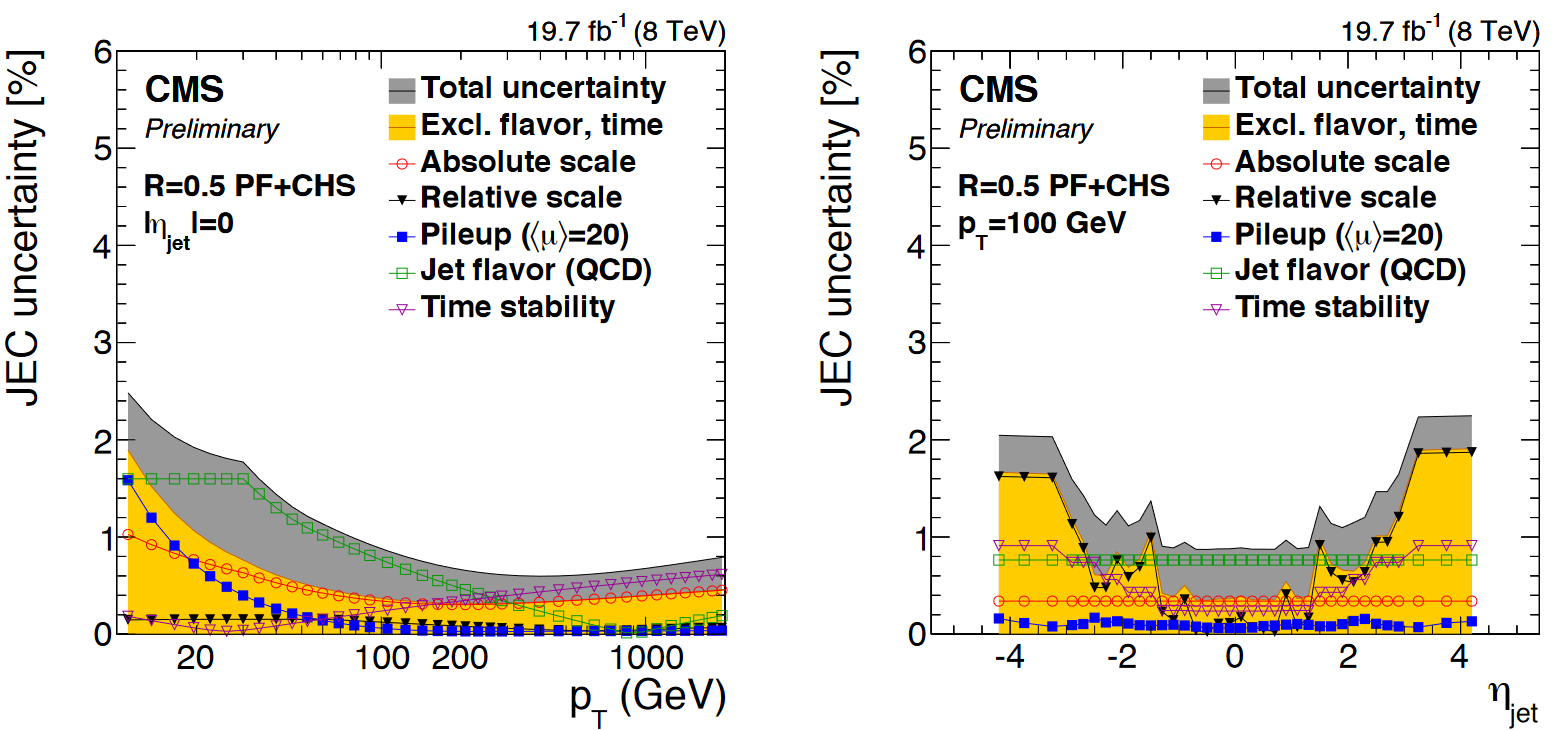# Jet Uncertainty¶

Unsurprisingly, the CMS detector does not measure jet energies perfectly, nor do simulation and data agree perfectly! The measured energy of jet must be corrected so that it can be related to the true energy of its parent particle. These corrections account for several effects and are factorized so that each effect can be studied independently.

## Jet Energy Corrections (JEC)¶

What is JEC?

JEC is the first set of corrections applied on jets that adjust the mean of the response distribution in a series of correction levels.

Correction LevelsParticles from additional interactions in nearby bunch crossings of the LHC contribute energy in the calorimeters that must somehow be distinguished from the energy deposits of the main interaction. Extra energy in a jet's cone can make its measured momentum larger than the momentum of the parent particle. The first layer ("L1") of jet energy corrections accounts for pileup by subtracting the average transverse momentum contribution of the pileup interactions to the jet's cone area. This average pileup contribution varies by pseudorapidity and, of course, by the number of interactions in the event.

The second and third layers of corrections ("L2L3") correct the measured momentum to the true momentum as functions of momentum and pseudorapidity, bringing the reconstructed jet in line with the generated jet. These corrections are derived using momentum balancing and missing energy techniques in dijet and Z boson events. One well-measured object (ex: a jet near the center of the detector, a Z boson reconstructed from leptons) is balanced against a jet for which corrections are derived.

All of these corrections are applied to both data and simulation. Data events are then given "residual" corrections to bring data into line with the corrected simulation. A final set of flavor-based corrections are used in certain analyses that are especially sensitive to flavor effects. All of the corrections are described in this paper. The figure below shows the result of the L1+L2+L3 corrections on the jet response.### Implementing JEC in CMS Software¶

JEC From Text Files

There are several methods available for applying jet energy corrections to reconstructed jets. We have demonstrated a method to read in the corrections from text files and extract the corrections manually for each jet. In order to produce these text files, we have to run jec_cfg.py.

``````isData = False
#if len(sys.argv) > 1: isData = bool(eval(sys.argv))
#print 'Writing JEC text files. isData = ',isData

# CMS process initialization
process = cms.Process('jecprocess')

# connect to global tag
if isData:
#    process.GlobalTag.connect = cms.string('sqlite_file:/cvmfs/cms-opendata-conddb.cern.ch/FT53_V21A_AN6_FULL.db')
process.GlobalTag.globaltag = 'FT53_V21A_AN6::All'
else:
#    process.GlobalTag.connect = cms.string('sqlite_file:/cvmfs/cms-opendata-conddb.cern.ch/START53_V27.db')
process.GlobalTag.globaltag = 'START53_V27::All'

process.maxEvents = cms.untracked.PSet(input=cms.untracked.int32(1))
process.source = cms.Source('EmptySource')
printScreen=cms.untracked.bool(False),
createTextFile=cms.untracked.bool(True))

if isData:
process.ak5.globalTag = cms.untracked.string('FT53_V21A_AN6')
else:
process.ak5.globalTag = cms.untracked.string('START53_V27')

process.p = cms.Path(process.ak5)
``````

Note that this analyzer will need to be run with both `isData = True` and `isData = False` to produce text files for both.

``````\$ cd JEC
\$ cmsRun jec_cfg.py
\$ #edit the file and flip isData
\$ cmsRun jec_cfg.py
``````

## Applying JEC Correction¶

JEC begins in poet_cfg.py, where we apply jet energy corrections and Type-1 MET corrections on PAT jets, which are a popular object format in CMS that stands for "Physics Analysis Toolkit". To do this we will load the global tag and databases directly in the configuration file and use the ‘addJetCollection’ process to create a collection of pat::jets.

Note: The JEC Uncertainty text file is needed for the manually created correction uncertainties created inside of the analyzer. Uncertainty will be covered later.

``````if doPat:
...
# Choose which jet correction levels to apply
jetcorrlabels = ['L1FastJet','L2Relative','L3Absolute']
if isData:
# For data we need to remove generator-level matching processes
runOnData(process, ['Jets','METs'], "", None, [])
jetcorrlabels.append('L2L3Residual')

# Set up the new jet collection
process.ak5PFJets.doAreaFastjet = True

'AK5', 'PFCorr',
doJTA        = True,
doBTagging   = True,
jetCorrLabel = ('AK5PF', cms.vstring(jetcorrlabels)),
doType1MET   = True,
doL1Cleaning = True,
doL1Counters = False,
doJetID      = True,
jetIdLabel   = "ak5",
)
process.myjets= cms.EDAnalyzer('PatJetAnalyzer',
InputCollection = cms.InputTag("selectedPatJetsAK5PFCorr"),
isData = cms.bool(isData),
jecUncName = cms.FileInPath('PhysObjectExtractorTool/PhysObjectExtractor/JEC/'+JecString+'Uncertainty_AK5PF.txt'),
jerResName = cms.FileInPath('PhysObjectExtractorTool/PhysObjectExtractor/JEC/JetResolutionInputAK5PF.txt')
)
...
``````

Now we can go into PatJetAnalyzer.cc, where in the jet loop of `analyzeJets`, the correction has already automatically been corrected for each jet. We then save a uncorrected version of the jet as `uncorrJet`.

``````for (std::vector<pat::Jet>::const_iterator itjet=myjets->begin(); itjet!=myjets->end(); ++itjet){
pat::Jet uncorrJet = itjet->correctedJet(0);
...
``````

## Jet Energy Resolution (JER)¶

What is JER?

Jet Energy Resolution (JER) corrections are applied after JEC on strictly MC simulations. Unlike JEC, which adjusts for the mean of the response distribution, JER adjusts the width of the distribution. This is because MC simulations tend to be more sharply peaked and less broad than the same distribution in data, therefore we have to increase the resolution based on the effects of pileup, jet size and jet flavor.

Accesing JER in CMS Software

Unlike JEC, the majority of JER is done inside of `PatJetAnalyzer.cc`, but we do have to import the file path to the text file containing a jet resolution factor table from the JEC directory in `poet_cfg.py`.

``````process.myjets= cms.EDAnalyzer('PatJetAnalyzer',
...
jerResName = cms.FileInPath('PhysObjectExtractorTool/PhysObjectExtractor/JEC/JetResolutionInputAK5PF.txt')
)
``````

Back inside the jet loop, we define `ptscale`, the eventual scale factor multiplied onto the jet momentum.

Note: As mentioned previously, if we are running `PatJetAnalyzer.cc` on data, we do not want to affect to the resolution, so we initialize it as `1`.

Next we calculate `ptscale` using one of two methods:

1. A stochastic smearing method, which is used on generator-level jets (`genJet`), described by equation 4.11 in this dissertation.

2. A hybrid smearing method, which is used otherwise, described in section 8 of the 2017 CMS jet algorithm paper, which also includes more information about JEC in general.

Note: Also mentioned previously was the fact that JER is applied after JEC, meaning the pT that is used various times in the evaluations (e.g `PTNPU.push_back( itjet->pt() );`) is the JEC corrected momentum, rather than the uncorrected one.

``````void
JetAnalyzer::analyze(const edm::Event& iEvent, const edm::EventSetup& iSetup)
{
...
for (std::vector<pat::Jet>::const_iterator itjet=myjets->begin(); itjet!=myjets->end(); ++itjet){
...
ptscale = 1;
res = 1;
if(!isData) {
std::vector<float> factors = factorLookup(fabs(itjet->eta())); // returns in order {factor, factor_down, factor_up}
std::vector<float> feta;
std::vector<float> PTNPU;
feta.push_back( fabs(itjet->eta()) );
PTNPU.push_back( itjet->pt() );
PTNPU.push_back( vertices->size() );

res = jer_->correction(feta, PTNPU);
float pt = itjet->pt();

const reco::GenJet *genJet = itjet->genJet();
bool smeared = false;
if(genJet){
double deltaPt = fabs(genJet->pt() - pt);
double deltaR = reco::deltaR(genJet->p4(),itjet->p4());
if ((deltaR < 0.2) && deltaPt <= 3*pt*res){
double gen_pt = genJet->pt();
double reco_pt = pt;
double deltapt = (reco_pt - gen_pt) * factors;
double deltapt_down = (reco_pt - gen_pt) * factors;
double deltapt_up = (reco_pt - gen_pt) * factors;
ptscale = max(0.0, (reco_pt + deltapt) / reco_pt);
...
smeared = true;
}
}
if (!smeared && factors>0) {
TRandom3 JERrand;

JERrand.SetSeed(abs(static_cast<int>(itjet->phi()*1e4)));
ptscale = max(0.0, JERrand.Gaus(pt,sqrt(factors*(factors+2))*res*pt)/pt);
...
}
``````

## Jet Correction Uncertainty¶

An important factor we have to keep in mind when applying both JEC and JER are the statistical uncertainities. These uncertainties have several sources, shown in the figure below. The L1 (pileup) uncertainty dominates at low momentum, while the L3 (absolute scale) uncertainty takes over for higher momentum jets. All corrections are quite precise for jets located near the center of the CMS barrel region, and the precision drops as pseudorapidity increases and different subdetectors lose coverage.These uncertainties are accounted for by including an "up" and "down" version of our correction factor.

JEC Uncertainty

While the JEC corrected momentum can be accessed automatically through the jet object (e.g. `itjet->pt()`), the "up" and "down" versions must be calculated manually.

Here in the jet loop, the `corrUp` and `corrDown` variables are created in part using `jetUnc_->getUncertainty()` (This object is created from the a text file which was briefly mentioned during the JEC initialization in `poet_cfg.py` of the Implementing JEC in CMS Software section). In order to access the `getUncertainty` function, we use a JEC uncertainty object, in this case called `jecUnc_`, where we input information about the jet, like its psuedorapidity and momentum.

``````for (std::vector<pat::Jet>::const_iterator itjet=myjets->begin(); itjet!=myjets->end(); ++itjet){
...
double corrUp = 1.0;
double corrDown = 1.0;
jecUnc_->setJetEta( itjet->eta() );
jecUnc_->setJetPt( itjet->pt() );
corrUp = (1 + fabs(jecUnc_->getUncertainty(1)));
jecUnc_->setJetEta( itjet->eta() );
jecUnc_->setJetPt( itjet->pt() );
corrDown = (1 - fabs(jecUnc_->getUncertainty(-1)));
...
``````

JER Uncertainty

Just how `ptscale` was manually calculated on genJets using this line:

``````ptscale = max(0.0, (reco_pt + deltapt) / reco_pt);
``````

We calculate the JER uncertainty like so:

``````ptscale_up = max(0.0, (reco_pt + deltapt_up) / reco_pt);
ptscale_down = max(0.0, (reco_pt + deltapt_down) / reco_pt);
``````

Otherwise for non-genJets,

``````JERrand.SetSeed(abs(static_cast<int>(itjet->phi()*1e4)));
ptscale_down = max(0.0, JERrand.Gaus(pt,sqrt(factors*(factors+2))*res*pt)/pt);

JERrand.SetSeed(abs(static_cast<int>(itjet->phi()*1e4)));
ptscale_up = max(0.0, JERrand.Gaus(pt,sqrt(factors*(factors+2))*res*pt)/pt);
``````

## Storing the corrections¶

The final step in actualizing the jet corrections occurs after the JEC/JER calculations, where we fill the five momentum vectors for each jet.

• `corr_jet_pt` is the JEC + JER corrected pT
• `corr_jet_ptUp` and `corr_jet_ptDown` are the ("up" and "down" versions of the JEC) + JER corrected pT
• `corr_jet_ptSmearUp` and `corr_jet_ptSmearDown` are the JEC + (smeared "up" and "down" versions of the JER) corrected pT
``````corr_jet_pt.push_back(ptscale*itjet->pt());
corr_jet_ptUp.push_back(ptscale*corrUp*itjet->pt());
corr_jet_ptDown.push_back(ptscale*corrDown*itjet->pt());
corr_jet_ptSmearUp.push_back(ptscale_up*itjet->pt());
corr_jet_ptSmearDown.push_back(ptscale_down*itjet->pt());
``````

## Putting it all together ¶

Inside of the dropdown is the full jet loop, comprised of the storing of the uncorrected jet object, creation of JEC uncertainty, JER corrections + uncertainty, and storing of the corrected momentum.

Full Jet Loop
``````for (std::vector<pat::Jet>::const_iterator itjet=myjets->begin(); itjet!=myjets->end(); ++itjet){
pat::Jet uncorrJet = itjet->correctedJet(0);

double corrUp = 1.0;
double corrDown = 1.0;
jecUnc_->setJetEta( itjet->eta() );
jecUnc_->setJetPt( itjet->pt() );
corrUp = (1 + fabs(jecUnc_->getUncertainty(1)));
jecUnc_->setJetEta( itjet->eta() );
jecUnc_->setJetPt( itjet->pt() );
corrDown = (1 - fabs(jecUnc_->getUncertainty(-1)));

ptscale = 1;
ptscale_down = 1;
ptscale_up = 1;
res = 1;
if(!isData) {
std::vector<float> factors = factorLookup(fabs(itjet->eta())); // returns in order {factor, factor_down, factor_up}
std::vector<float> feta;
std::vector<float> PTNPU;
feta.push_back( fabs(itjet->eta()) );
PTNPU.push_back( itjet->pt() );
PTNPU.push_back( vertices->size() );

res = jer_->correction(feta, PTNPU);
float pt = itjet->pt();

const reco::GenJet *genJet = itjet->genJet();
bool smeared = false;
if(genJet){
double deltaPt = fabs(genJet->pt() - pt);
double deltaR = reco::deltaR(genJet->p4(),itjet->p4());
if ((deltaR < 0.2) && deltaPt <= 3*pt*res){
double gen_pt = genJet->pt();
double reco_pt = pt;
double deltapt = (reco_pt - gen_pt) * factors;
double deltapt_down = (reco_pt - gen_pt) * factors;
double deltapt_up = (reco_pt - gen_pt) * factors;
ptscale = max(0.0, (reco_pt + deltapt) / reco_pt);
ptscale_up = max(0.0, (reco_pt + deltapt_up) / reco_pt);
ptscale_down = max(0.0, (reco_pt + deltapt_down) / reco_pt);
smeared = true;
}
}
if (!smeared && factors>0) {
TRandom3 JERrand;

JERrand.SetSeed(abs(static_cast<int>(itjet->phi()*1e4)));
ptscale = max(0.0, JERrand.Gaus(pt,sqrt(factors*(factors+2))*res*pt)/pt);

JERrand.SetSeed(abs(static_cast<int>(itjet->phi()*1e4)));
ptscale_down = max(0.0, JERrand.Gaus(pt,sqrt(factors*(factors+2))*res*pt)/pt);

JERrand.SetSeed(abs(static_cast<int>(itjet->phi()*1e4)));
ptscale_up = max(0.0, JERrand.Gaus(pt,sqrt(factors*(factors+2))*res*pt)/pt);
}
}

if( ptscale*itjet->pt() <= min_pt) continue;

jet_pt.push_back(uncorrJet.pt());
jet_eta.push_back(itjet->eta());
jet_phi.push_back(itjet->phi());
jet_ch.push_back(itjet->charge());
jet_mass.push_back(uncorrJet.mass());
jet_btag.push_back(itjet->bDiscriminator("combinedSecondaryVertexBJetTags"));
corr_jet_pt.push_back(ptscale*itjet->pt());
corr_jet_ptUp.push_back(ptscale*corrUp*itjet->pt());
corr_jet_ptDown.push_back(ptscale*corrDown*itjet->pt());
corr_jet_ptSmearUp.push_back(ptscale_up*itjet->pt());
corr_jet_ptSmearDown.push_back(ptscale_down*itjet->pt());
corr_jet_mass.push_back(itjet->mass());
corr_jet_e.push_back(itjet->energy());
corr_jet_px.push_back(itjet->px());
corr_jet_py.push_back(itjet->py());
corr_jet_pz.push_back(itjet->pz());
...
}
``````